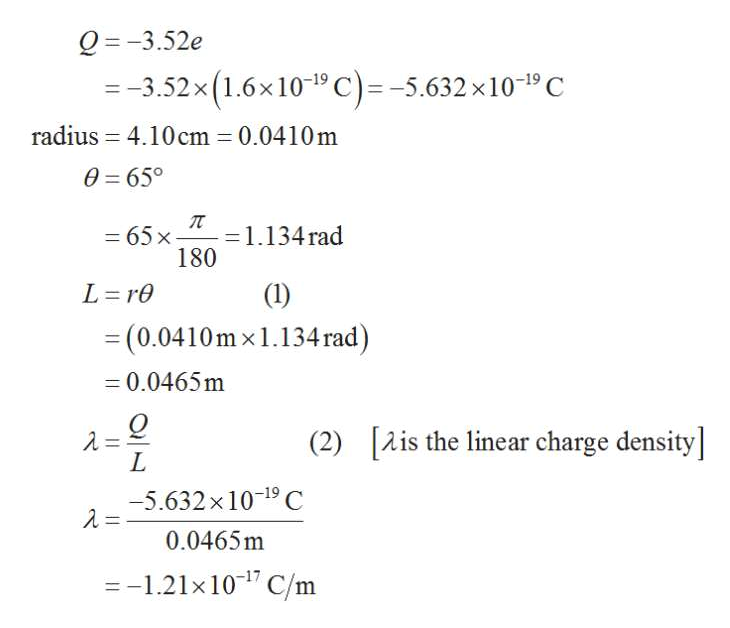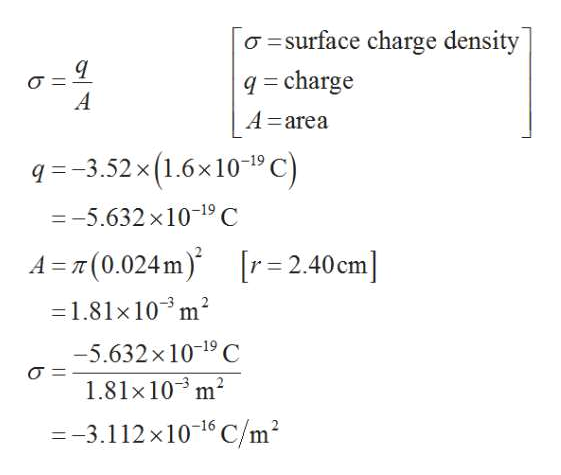Density, density, density. (a) A charge -352e is uniformly distributed along a circular arc of radius 4.10 cm, which subtends an angle of 65o. What is the linear charge density along the arc? (b) A charge -352e is uniformly distributed over one face of a circular disk of radius 2.40 cm. What is the surface charge density over that face? (c) A charge -352e is uniformly distributed over the surface of a sphere of radius 5.20 cm. What is the surface charge density over that surface? (d) A charge -352e is uniformly spread through the volume of a sphere of radius 3.10 cm. What is the volume charge density in that sphere?

Question

Density, density, density. (a) A charge -352e is uniformly distributed along a circular arc of radius 4.10 cm, which subtends an angle of 65o. What is the linear charge density along the arc? (b) A charge -352e is uniformly distributed over one face of a circular disk of radius 2.40 cm. What is the surface charge density over that face? (c) A charge -352e is uniformly distributed over the surface of a sphere of radius 5.20 cm. What is the surface charge density over that surface? (d) A charge -352e is uniformly spread through the volume of a sphere of radius 3.10 cm. What is the volume charge density in that sphere?

Step 1

Hey, since there are multiple subparts posted, we will answer first three subparts. If you want any specific subpart to be answered then please submit that question only or specify the question number in your message.

Step 2

(a) The linear charge density along the arc is the total charge divided by the length of the arc. It is the measure of a quantity of any characteristic value per unit length.help_outlineImage TranscriptioncloseQ-3.52e =-3.52x (1.6x1019 C)=-5.632 x10-19 C radius 4.10cm 0.0410m 0 65° =1.134rad 180 -65x L re (1) =(0.0410m x 1.134rad) = 0.0465m Q [Ais the linear charge density] (2) -5.632x 1019 C 0.0465m =-1.21x101 C/m fullscreen
Step 3

(b) Surface charge density present only in conducting surfaces a...help_outlineImage Transcriptioncloseasurface charge density q=charge A A=area q-3.52x (1.6x101C =-5.632 x 1019 C A (0.024 m [r= 2.40cm] 1.81x 103m2 -5.632x 1019 C 1.81x 10 m2 -3.112x1016 C/m2 fullscreen

Want to see the full answer?

See Solution

Want to see this answer and more?

Our solutions are written by experts, many with advanced degrees, and available 24/7

See Solution
Tagged in

Electric Charges and Fields